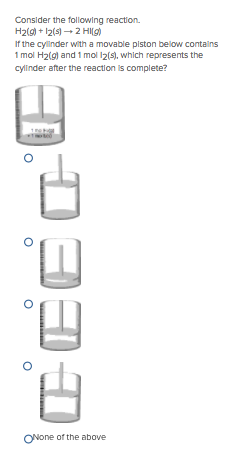# Problem: Consider the following reaction. H2 (g) + I2 (s) → 2HI (g) If the cylinder with a movable piston below contains 1 mol H2(g) and 1 mol I2(s), which represents the cylinder after the reaction is complete?

###### FREE Expert Solution
90% (186 ratings)###### Problem Details

Consider the following reaction. H(g) + I(s) → 2HI (g)

If the cylinder with a movable piston below contains 1 mol H2(g) and 1 mol I2(s), which represents the cylinder after the reaction is complete?